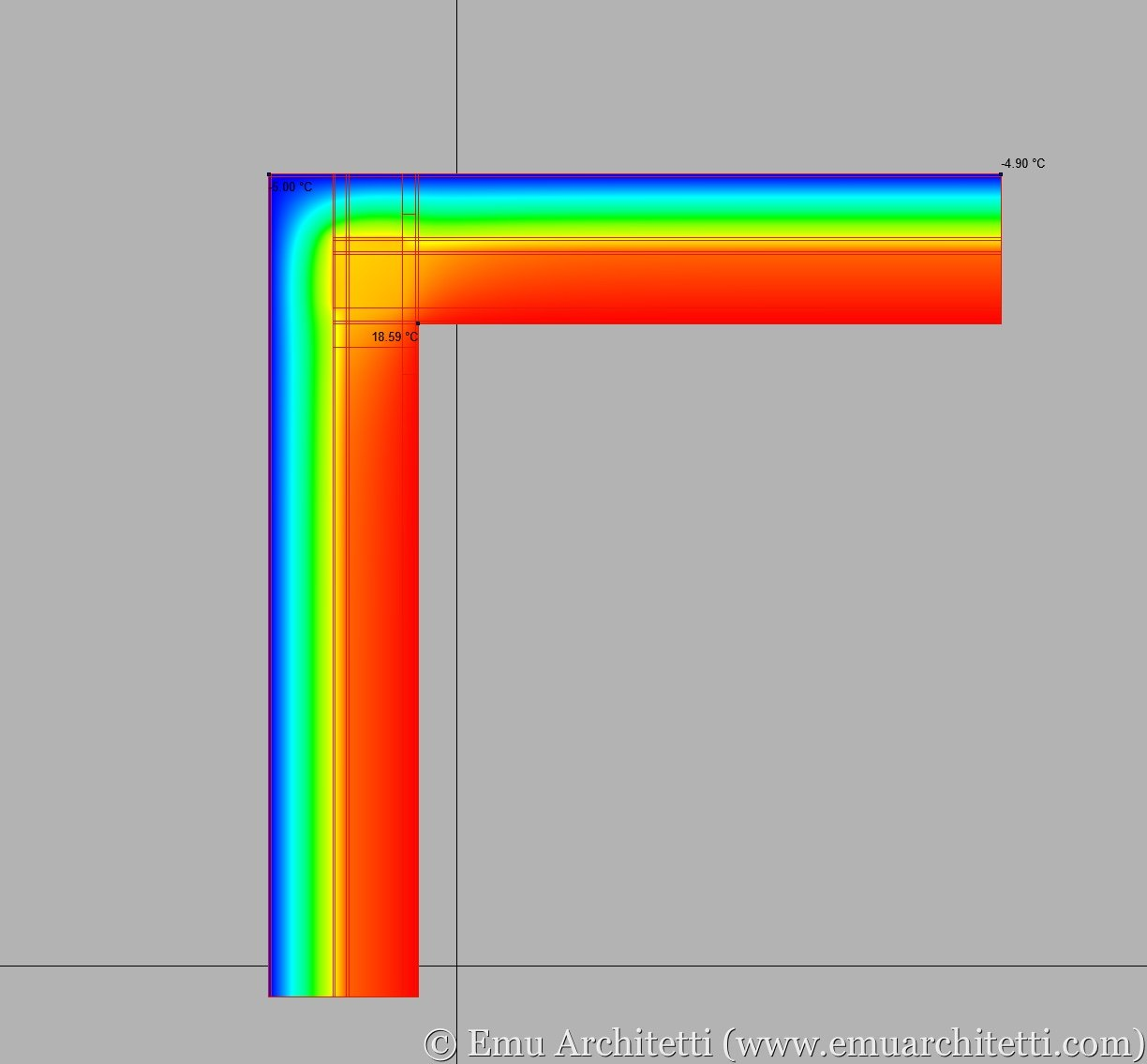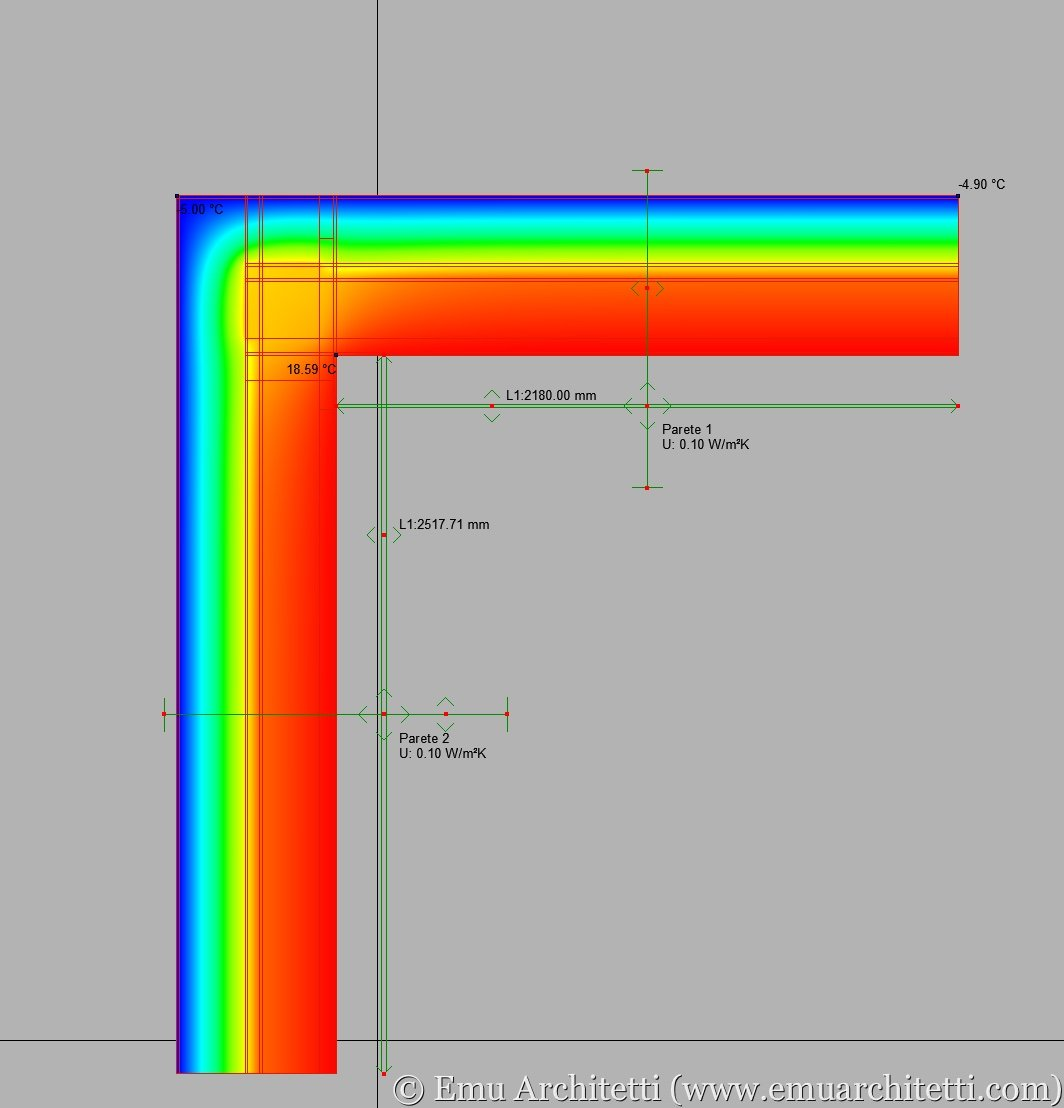# Thermal bridges: the PSI value

For new builds as well as energy retrofits, the construction industry worldwide is shifting more and more towards zero energy buildings and Passive Houses.

With the thermal envelope becoming more and more advanced, the correct evaluation of thermal bridges becomes critical: in this article, we explain the PSI value.

We’re going to address the role of thermal bridges as far as comfort, including mold and condensation, in a separate article.

## PARAMETERS

When you develop the energy design of a building, the first step to take is to determine the energy needed to keep the internal environment comfortable. It is called “net energy demand” because it does not take into account the building systems (including renewable energy sources). Only the following parameters are considered:

• thermal coupling between the structures of the building (including windows) and the outside;
• heat losses/gains via ventilation, including air leaks;
• solar heat gains — the “passive” heating received through glazed components;
• interior heat gains, provided by body heat and appliances.

The reference norm is ISO 13790, which is the base of PHPP, the calculation tool to design passive buildings. In the list above, thermal bridges are included in the first point.

In order to understand what a PSI value means, you need to understand how the thermal coupling between inside and outside is calculated.

The heat migrates spontaneously from a warmer body to a colder one, following the “easiest” path available. After all, heat is “lazy” enough to be predictable. In the real world, the heat flows through the structures of the thermal envelope following bidimensional or tridimensional paths.The bi-dimensional heat flows through the structures of the thermal envelope — in this case, at a corner of the building.

When you design a building, however, the heat flow is calculated as if the heat would follow a straight line (mono-dimensional flow) from the warm side of the structure to the cold one. This is the bottom line of ISO 6946, which dictates how you calculate the thermal transmittance and R-value of an opaque structure.

The heat flow calculated in a mono-dimensional way is different from the one that occurs in reality (bidimensional or tridimensional heat flow). The difference between the two flows is the PSI value.

You need to be careful: the same thermal bridges can have different PSI values, depending on how the mono-dimensional flow is calculated.

We include here a double example, showing how the PSI value changes depending on whether the mono-dimensional flow is calculated according to the inside measurements of the structures, or to the outside ones (also see ISO 13789).

## EXAMPLE: CALCULATING WITH INTERNAL MEASUREMENTS

Calculation according to internal measurements — see the green arrows in the image below — is used to determine the heat load of individual rooms.The thermal bridge calculated according to the internal measurements, here represented by the green arrows in the picture.

The bidimensional heat flow — calculated with a finite element software according to ISO 10211 — is the following L2D value:

Bidimensional flow L2D: 0,5716 W/mK (0,3303 BTU/(h*ft*°F))

The mono dimensional heat flow, calculated according to the internal measurements, is calculated as follows:

Wall #1: 2,18 m * 0,103 W/m2K = 0,2245 W/mK (7,15 ft * 0,0181 BTU/(h*ft2*°F) = 0,1294 BTU/(h*ft*°F))

Wall #2: 2,57 m * 0,103 W/m2K = 0,2647 W/mK  (8,43 ft* 0,0181 BTU/(h*ft2*°F) = 0,1526 BTU/(h*ft*°F))

Total mono dimensional flow (L1D), internal measurements: 0,2245 + 0,2647 = 0,4892 W/mK. (0,2820 BTU/(h*ft*°F))

The difference between the bidimensional and mono dimensional heat flows , according to the internal measurements, is PSI-i:

PSI-i = L2D – L1D = 0,5716 – 0,4892 = 0,0824 W/mK. (0,3303 – 0,2820 = 0,0483 BTU/(h*ft*°F))

## EXAMPLE: CALCULATING WITH EXERNAL MEASUREMENTS

Calculations according to the external measurements are used to evaluate the whole thermal envelope, as in the case of PHPP, because the results are more conservative.

The bidimensional flow is exactly the same as the example above — it would only change if we were to modify the geometry of the node, or the materials.

Bidimensional flow (L2D): 0,5716 W/mK (0,3303 BTU/(h*ft*°F));

The mono dimensional flow L1D changes, because this time we are calculating it according to the external measurements:

Wall #1: 2,74 m * 0,103 W/m2K = 0,2822 W/mK (8,99 ft * 0,0181 BTU/(h*ft2*°F) = 0,1627 BTU/(h*ft*°F))

Wall #2: 3,08 m * 0,103 W/m2K = 0,3172 W/mK (10,10 ft * 0,0181 BTU/(h*ft*°F) = 0,1828 BTU/(h*ft*°F))

Total mono dimensional flow (L1D), external measurements: 0,2822 + 0,3172 = 0,5994 W/mK (0,1627 + 0,1828 = 0,3455 BTU/h*ft*°F))

The difference between bidimensional and mono dimensional flows, according to the external measurements, is PSI-e:

PSI-e: L2D – L1D = 0,5716 – 0,5994 = -0,0278 W/mK (0,3303 – 0,3455 = -0,0152 BTU/(h*ft*°F))

## NEGATIVE PSI VALUES

The numerical value of PSI is calculated via the arithmetic subtraction between the bidimensional flow L2D and the mono-dimensional one L1D: if the numerical value of L1D is higher than the one of L2D, as in the example above, the value of PSI is lower than zero. In terms of heat flow, a “negative” value of PSI has only a mathematical meaning: it does not express a value for the quality of the construction it refers to.

## CONCLUSIONS

The double example above is useful to explain what the PSI value represents. It is just a number used to match the mono-dimensional flow to the bi-dimensional one. The numerical value of PSI changes based on how the overall heat calculation of the building is set up — either according to the internal measurements or to the external ones.

The same thermal bridge has different values of PSI, depending on how the building is calculated.

If you don’t know what the PSI value refers to, you cannot say whether it is “high” or “low.”

The PSI value cannot stand alone: it necessarily refers to specific structures and geometry.

If you want to compare the PSI values of different design solutions, you can only do it if these values are calculated in the same way.